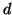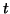Next: Demos Up: Utility Scripts Previous: perlgp-mrun.pl   Contents

## perlgp-avg-logs.pl

When you've run 50 copies of the same job using perlgp-mrun.pl, this script will take the last line of each `results/tournament.log' file and calculate the means and standard deviations from each numeric column. For non-numeric columns it prints the most common string seen in that column.

```  usage: perlgp-avg-logs.pl label1 'glob1-??' label2 'glob2-??' label3...
```

Where each label is an arbitrary identifier for the experiments which the following shell glob (protected in quotes) expands to. Perhaps this is best explained with an example. Imagine you are running two symbolic regression experiments, one with trigonometric functions, one without. Assuming you ran perlgp-mrun.pl on two experiments named `fit-withtrig' and `fit-notrig', then you would use this program as follows:

```  example: perlgp-avg-logs.pl notrig 'fit-notrig-??' trig 'fit-withtrig-??'
```

When exactly two sets of experiments are given, as above, this program also prints out thevalue from a paired-test (asks if the means from two assumed normal distributions are significantly different). Look this up in any statistics text book, but as a rough guide, if you have done more than 30 replicates of each experiment and the absolute value ofis greater than 1.96, then the two means are significantly different at the 5% level.

If you want to do the averaging at a particular tournament number, and not on the final tournament, add the option -tournament T, where T is the tournament number. If this tournament is not in the logfile, the last entry in the log is used.

You can specify logarithm-taking of certain columns in the log file with -logs COL1 -logs COL2 or -logs 1,5 and set the log base with -base N. Column numbers start at zero.Next: Demos Up: Utility Scripts Previous: perlgp-mrun.pl   Contents
Bob MacCallum 2003-02-03